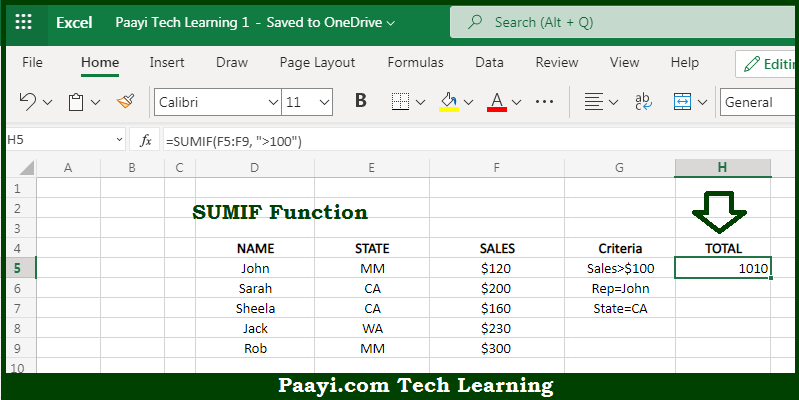# Learn How to Use Microsoft Excel SUMIF Function

Written by | 0 Comments | 628 Views

In this article, you will learn how to use the Microsoft Excel SUMIF function and its prime function in Microsoft Excel. You will also get to know the Microsoft Excel SUMIF function return value and syntax with the help of some examples.

Microsoft Excel SUMIF Function

The main function of the Microsoft Excel SUMIF function is to sum the numbers in a range that meet the required criteria. That implies, with the help of SUMIF function you can able to return the sum of cells that meet the single condition. It should be noted that criteria can be applied to dates, numbers, and text. The SUMIF function also supports logical operators like (>,<,<>,=) and wildcards (*,?) for partial matching. So, with the help of the SUMIF function, you can able to sum the numbers in a range that meet the required criteria.

Return Value of SUMIF Function

The return value will be the sum of values supplied.

Syntax of SUMIF Function

=SUMIF(range, criteria, [sum-range])

Where the arguments:

• range: This is the range of cells that you want to apply the criteria against.
• criteria: It is the criteria used to determine which cells to add.
• sum-range: This is the cells to add together. If the sum range is omitted, the cells in the range are added together instead. (optional).

## How to Use Microsoft Excel SUMIF Function?So we know that Microsoft Excel SUMIF function you can able to sum the numbers in a range that meets the required criteria. That implies, with the help of SUMIF function you can able to return a sum of cells that meet the single condition. It should be noted that criteria can be applied to dates, numbers, and text. The SUMIF function also supports logical operators like (>,<,<>,=) and wildcards (*,?) for partial matching. So, with the help of the SUMIF function, you can able to sum the numbers in a range that meet the required criteria.